### Home > INT1 > Chapter Ch8 > Lesson 8.1.1 > Problem8-7

8-7.
1. Write the multiplier for each increase or decrease described below. Homework Help ✎

1. A 25% increase

2. A decrease of 18%

3. An increase of 39%

4. A decrease of 94%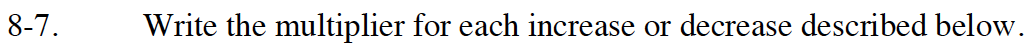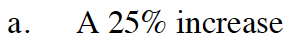See Math Notes Box in Lesson 5.3.2 to understand the idea of multipliers.

To keep a value the same you multiply by 1.00, to increase a value the multiplier must be greater than 1.00. If we are increasing by 25% (0.25) what should the multiplier be?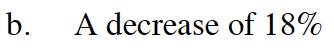The multiplier is 0.82.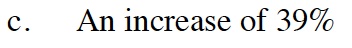See part (a).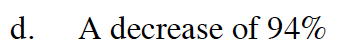See part (a).